LC circuits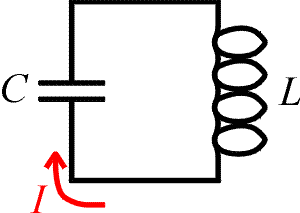An LC circuit is simply a closed loop with only two elements, a capacitor and an inductor. We will show that the LC circuits have resonant properties - they respond to certain frequencies. Therefore they serve as the basis for any device that needs to tune to a specific frequency such as a radio. In an LC loop the charge oscillates back and forth through the capacitor with a given frequency just as a mass on a spring oscillates. To demonstrate the equivalence, we list several equations for both a mechanical spring and for an LC circuit.

 mechanical spring LC circuit velocity:current: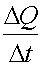kinetic energy: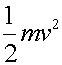inductor's energy: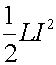potential energy: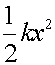capacitor's energy: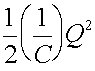Eq. of motion: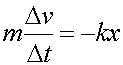Kirchoff's law: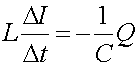frequency: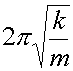frequency: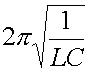The parameters that determine the motion of a spring are the mass m, spring constant k, the position x, and the velocity v which is the rate of change of x. The parameters that determine the behavior of an LC circuit are L, C, Q and I which is the rate of change of x. Thus there is a one-to-one correspondence since the equations of motion are identical given the substitutions: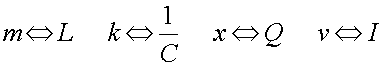The characteristic frequency of and LC circuit is the frequency at which large amplitudes are built up when a driving force comes in at that frequency. A child on a swing will be sensitive to a pushing force which comes regularly with the natural frequency of the swing. A force that comes at a different frequency will not build up a large amplitude as it will often be pushing against the child's motion. We call this the resonant frequency.